##帐号 自动登录 找回密码 密码 立即注册→加入我们
 搜索 热搜: 下载 VB C 实现 编写

# 【Python】高斯消元法与LU分解29主题 1999积分

UID
3808

11

105 点

1238 个

165 次

0 份

378 小时

2018-5-6发表于 2020-9-22 20:37:21 | 显示全部楼层 |阅读模式

### 欢迎访问技术宅的结界，请注册或者登录吧。

x

1.系数矩阵的各阶顺序主子式均不为零。
2.系数矩阵是严格的对称正定矩阵
3.系数矩阵是严格的对角占优矩阵

[Python] 纯文本查看 复制代码
#!/usr/bin/env python
#! -*- coding:UTF-8 -*-
# 日期: 2020/9/18
# 作者: 周星光
# 组织: XJTU NuTHeL
# 备注: 今天是九一八，勿忘国耻，共勉。

# 高斯消元法
# 本程序不适用任何第三方库(如NumPy等)，保持上课学习计算方法的原汁原味，
# 当使用NumPy时，可以直接利用其中的向量操作，所以在高斯消元时可以少写一个内层循环。
# 综上，也就是说当您阅读本程序时，会耗费更多的脑力，如果您今天感觉不适，请立即关闭电脑进行休息。

# 导入数学库，使用其中的fabs绝对值函数
import math

# 定义一个极小值
e = 0.00001

# 打印矩阵
def ShowMatrix(matrix):
print("=================")
row = len(matrix)
# 按行打印
for i in range(row):
print(matrix[i][:])
print("=================")

# 原生的二维矩阵运算函数
def GaussMethod(matrix, b):
"""
matrix为系数矩阵，需要求解的矩阵
b为方程等号右边的列向量，需要同步求解
返回值为求解结果x
"""
# 矩阵的行数
row = len(matrix)
# 矩阵的列数
col = len(matrix)
# 定义用于存储结果的向量，默认结果全部为0
x =  * col
# 将matrix系数矩阵转化为上三角矩阵
for i in range(row-1): # len(matrix)用于求解matrix矩阵的行数
# 如果程序中主对角线元素近似于0，
# 则说明该方程组的求解不适合使用高斯消元法，应当考虑列主元高斯消元法。
if math.fabs(matrix[i][i]) <= e:
print("Please recheck the triangle element, which maybe near zero...")
return None
for j in range(i+1, row):
# 求解变换系数
coeff = matrix[j][i] / matrix[i][i]
# 开始进行行变换
for k in range(col): # len(matrix) 用于求出矩阵的列数
matrix[j][k] = matrix[j][k] - coeff * matrix[i][k]
# 等号右边的列变量同样也需要进行对应的行变换
b[j] = b[j] - coeff * b[i]

# 打印高斯消元后的临时结果
ShowMatrix(matrix)
print(b)

###############################
# 接下来进行回代过程
###############################
# 先求列向量x的最后一个元素的值
x[len(x)-1] = b[col-1] / matrix[col-1][col-1]
for i in range(row-2, -1, -1):
for j in range(i, col-1):
b[i] = b[i] - matrix[i][j+1] * x[j+1]
x[i] = b[i] / matrix[i][i]

return x

# 由于高斯消元法的应用条件比较苛刻，所以可以考虑更加具有普适性的列主元高斯消元法
def ColMaxGaussMethod(matrix, b):
"""
列主元高斯消元法
"""
# 矩阵的行数
row = len(matrix)
# 矩阵的列数
col = len(matrix)
# 存储方程组的解
x =  * col
# 进行第k次消元
for k in range(row-1):
# 开始选取列主元
max_number = 0
index = -1
for i in range(k, row):
# 注意此处进行比较的时绝对值
if math.fabs(matrix[i][k]) > math.fabs(max_number):
max_number = matrix[i][k]
index = i # 记录是第几行的元素
# 当这一列的主元为一个接近零的极小值时，退出程序，因为这个方程组无法进行求解。
if math.fabs(max_number) <= e:
print("WARNING! Please recheck the formula and identify it can be solved!")
return None
# 如果发现列上的最大元素不是当前对角线上的元素，
# 则进行行交换
if index != k:
# 行交换
for i in range(col):
matrix[k][i], matrix[index][i] = matrix[index][i], matrix[k][i]
# 等号右边的列向量同样需要进行行变换操作。
b[k], b[index] = b[index], b[k]
# 第k次消元前选列主元，交换列主元行变换完毕。
# 下面开始进行当前行的高斯消元
for j in range(k+1, row):
# 求解变换系数
coeff = matrix[j][k] / matrix[k][k]
for i in range(col):
matrix[j][i] = matrix[j][i] - coeff * matrix[k][i]
# 等号右边的列变量同样也需要进行对应行变换
b[j] = b[j] - coeff * b[k]

# 打印高斯消元后的临时结果
ShowMatrix(matrix)
print(b)
# 高斯消元完毕后，进行回代过程
# 先求列向量x的最后一个元素的值
x[len(x)-1] = b[col-1] / matrix[col-1][col-1]
for i in range(row-2, -1, -1):
for j in range(i, col-1):
b[i] = b[i] - matrix[i][j+1]*x[j+1]
x[i] = b[i] / matrix[i][i]

return x

if __name__ == '__main__':
test_a = [[1, 2, 1], [2, 2, 3], [-1, -3, 0]]
b = [0, 3, 2]
result = GaussMethod(test_a, b)
print('result=', result)

test_b = [[2, 2, 3], [1, 2, 1], [-1, -3, 0]]
b = [3, 0, 2]
print('测试列主元高斯消元法')
result = ColMaxGaussMethod(test_b, b)
print('result=', result)

test_c = [[1, 2, 1], [2, 2, 3], [-1, -3, 0]]
b = [0, 3, 2]
print('测试列主元高斯消元法')
result = ColMaxGaussMethod(test_c, b)
print('result=', result)

=================
[1, 2, 1]
[0.0, -2.0, 1.0]
[0.0, 0.0, 0.5]
=================
[0, 3.0, 0.5]
result= [1.0, -1.0, 1.0]

=================
[2, 2, 3]
[0.0, -2.0, 1.5]
[0.0, 0.0, 0.25]
=================
[3, 3.5, 0.25]
result= [1.0, -1.0, 1.0]

=================
[2, 2, 3]
[0.0, -2.0, 1.5]
[0.0, 0.0, 0.25]
=================
[3, 3.5, 0.25]
result= [1.0, -1.0, 1.0]

[Python] 纯文本查看 复制代码
#! /usr/bin/env python
#! -*- coding:utf-8 -*-
# Author : Xingguang Zhou
# Date   : 2020/9/22
# Program: 矩阵的LU分解
[color=Red]# 更改的内容：2020/9/26在之前的计算方法中，由于上课和书上的例子全部都是对称矩阵（即原矩阵与其转置矩阵相等）
# 所以在计算方法的理解上出现了小的偏差，但是最终结果是正确的，所以在和刘洪权同学的讨论中，
# 他非常细心地给我指正了错误，并帮助我修改了程序，非常感谢他！
# 需要注意的是，LU分解中，L矩阵依旧需要像U矩阵的求解那样，进行二重循环求解。[/color]

# Print the matrix
def PrintMatrix(matrix):
print('===================')
row = len(matrix)
for _ in range(row):
print(matrix[_])
print('===================')

# LU decomposition matrix
# Input  : An original matrix which need to be decomposed in LU method.
# Return : L matrix and U matrix
def LU(matrix):
# The row number of the matrix
row = len(matrix)
# The coloumn number of the matrix
col = len(matrix)

""" Caution! We assume the row is equal to col, so this matrix is a square matrix. """

# Use the list comprehension
# Initial the U matrix
U = [[0 for i in range(row)] for j in range(col)]
# Initial the L matrix
L = [[0 for i in range(row)] for j in range(col)]

# The first frame
for i in range(col):
U[i] = matrix[i]
L[i] = matrix[i] / matrix

# 开始进行第二框及以后的LU分解。
for i in range(1, row):
# 这是我写的方法，运算量比书上的小多了。
# 开始处理非对角线上的元素
for j in range(i, col):
# 开始计算U矩阵的第i行
summary = 0
for k in range(i):
summary = summary + U[k][j] * L[i][k]
U[i][j] = matrix[i][j] - summary
# 下面开始计算L矩阵第i列
summary = 0
for k in range(j):
summary = summary + U[k][i] * L[j][k]
L[j][i] = (matrix[j][i] - summary) / U[i][i]

PrintMatrix(U)
PrintMatrix(L)

if __name__ == '__main__':
print("第一次实验：")
matrix = [[ 4,  -2,   0,  4],
[-2,   2,  -3,  1],
[ 0,  -3,  13, -7],
[ 4,   1,  -7, 23]]
LU(matrix)

print("第二次实验：")
matrix = [[  9,  18,   9, -27],
[ 18,  45,   0, -45],
[  9,   0, 126,   9],
[-27, -45,   9, 135]]
LU(matrix)

print("第三次实验：")
matrix = [[2, 2, 2],
[4, 7, 7],
[6, 18, 22]]
LU(matrix)

===================
[4, -2, 0, 4]
[0, 1.0, -3.0, 3.0]
[0, 0, 4.0, 2.0]
[0, 0, 0, 9.0]
===================
===================
[1.0, 0, 0, 0]
[-0.5, 1.0, 0, 0]
[0.0, -3.0, 1.0, 0]
[1.0, 3.0, 0.5, 1.0]
===================

===================
[9, 18, 9, -27]
[0, 9.0, -18.0, 9.0]
[0, 0, 81.0, 54.0]
[0, 0, 0, 9.0]
===================
===================
[1.0, 0, 0, 0]
[2.0, 1.0, 0, 0]
[1.0, -2.0, 1.0, 0]
[-3.0, 1.0, 0.6666666666666666, 1.0]
===================

===================
[2, 2, 2]
[0, 3.0, 3.0]
[0, 0, 4.0]
===================
===================
[1.0, 0, 0]
[2.0, 1.0, 0]
[3.0, 4.0, 1.0]
===================

### 评分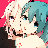0xAA55 + 10 + 20 + 30 啊！python
Passion Coding！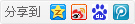1088主题 7万积分

UID
1

236

474 点

21358 个

45937 次

0 份

2058 小时

2014-1-26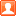发表于 2020-9-22 23:25:16 | 显示全部楼层
 我完全不会数学

29主题 1999积分

UID
3808

11

105 点

1238 个

165 次

0 份

378 小时

2018-5-6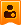楼主| 发表于 2020-9-25 18:50:54 | 显示全部楼层
 0xAA55 发表于 2020-9-22 23:25 我完全不会数学 哈哈，感谢站长的鼓励！ 怎么说呢，小弟我是工科的，然后就是掌握一种方法来应用，至于“会数学”这种事情，我也不是很会数学，老师讲，我想听就听了，然后把自己理解的，写成一个帖子来分享一下，是我所愿意的； 此外，小弟的工科数学主要是针对方程组的求解，积分的运算，微分方程离散为差分方程的求解，和图形学中的向量相关的数学还是有一些区别的，所以在向量、空间坐标系等方面的知识还是有所欠缺，所以请多多指教！
Passion Coding！

29主题 1999积分

UID
3808

11

105 点

1238 个

165 次

0 份

378 小时

2018-5-6楼主| 发表于 2020-9-27 09:41:02 | 显示全部楼层
 矩阵的三对角矩阵的“追赶法”，依旧使用LU分解，三对角矩阵的LU分解非常的简单，运算步骤也非常的少， 进行完毕LU分解之后，先利用Ly = d求出中间过程的解向量y，之后再带入Ux = y中求出最终的解向量x 代码如下： [AAuto] 纯文本查看 复制代码 ! 追赶法分解三对角矩阵 module TridiagonalMatrix implicit none ! 本结构体在该程序中用处不大，值得一提的是， ! 在稀疏矩阵的运算过程中，可以使用该结构体来记录那些不为零的元素。 type :: point integer :: i integer :: j real :: value end type contains ! 为了使用矩阵的列相关操作，举例：定义matrix(3, 4)为4行3列矩阵 subroutine PrintMatrix(matrix) implicit none real, intent(in) :: matrix(:, :) integer :: i integer :: row row = size(matrix, 2) ! 按行打印矩阵 do i=1, row write(*, *) matrix(:, i) end do return end subroutine ! 开始进行追赶法进行LU分解 subroutine Run_Chase(matrix, L, U, b) implicit none real, intent(in) :: matrix(:, :) real, intent(out) :: L(:, :) real, intent(out) :: U(:, :) real, intent(inout) :: b(:) ! 方程等号右边的列矩阵 real, allocatable :: y(:) integer :: i, j integer :: row integer :: col row = size(matrix, 2) ! 为中间方程结果y申请内存空间 allocate(y(row)) ! 先计算矩阵的第一个数 U(1, 1) = matrix(1, 1) L(1, 1) = 1.0 y(1) = b(1) ! 开始进行L和U的求解 do i=2, row L(i-1, i) = matrix(i-1, i) / U(i-1, i-1) L(i, i) = 1 U(i, i) = matrix(i, i) - L(i-1, i) * matrix(i, i-1) U(i, i-1) = matrix(i, i-1) y(i) = b(i) - L(i-1, i) * y(i-1) end do ! 将y计算的值返回给b b = y ! 释放y申请的空间 deallocate(y) return end subroutine ! 利用高斯消元法中的回代过程完成方程组的最终求解 subroutine Solution(U, b, x) implicit none real, intent(inout) :: U(:, :) real, intent(inout) :: b(:) real, intent(out) :: x(:) ! 用于存放最终结果 integer :: row, col, i, j row = size(U, 2) col = size(U, 1) x(row) = b(row) / U(row, row) do i=row-1, 1, -1 do j=col, i+1, -1 b(i) = b(i) - U(j, i) * x(j) end do x(i) = b(i) / U(i, i) end do return end subroutine end module ! 开始主程序 program main use TridiagonalMatrix implicit none integer :: i, j real :: matrix(5, 5) = (/ 1, 2, 0, 0, 0, 2, 3, 1, 0, 0, 0, -3, 4, 2, 0, 0, 0, 4, 7, 1, 0, 0, 0, -5, 6 /) real :: L(5, 5) = 0 real :: U(5, 5) = 0 real :: b(5) = (/ 5, 9, 2, 19, -4 /) real :: x(5) = 0 integer :: row = 5 call Run_Chase(matrix, L, U, b) write(*, *) "三对角矩阵的LU分解如下所示：" write(*, *) "==============================L矩阵==============================" call PrintMatrix(L) write(*, *) "==============================U矩阵==============================" call PrintMatrix(U) write(*, *) "============================中间b向量=============================" write(*, *) b ! 解方程 call Solution(U, b, x) write(*, *) "方程的最终结果：" write(*, *) x end program  运行结果： 三对角矩阵的LU分解如下所示： ==============================L矩阵==============================    1.000000      0.0000000E+00  0.0000000E+00  0.0000000E+00  0.0000000E+00    2.000000       1.000000      0.0000000E+00  0.0000000E+00  0.0000000E+00   0.0000000E+00   3.000000       1.000000      0.0000000E+00  0.0000000E+00   0.0000000E+00  0.0000000E+00   4.000000       1.000000      0.0000000E+00   0.0000000E+00  0.0000000E+00  0.0000000E+00   5.000000       1.000000 ==============================U矩阵==============================    1.000000       2.000000      0.0000000E+00  0.0000000E+00  0.0000000E+00   0.0000000E+00  -1.000000       1.000000      0.0000000E+00  0.0000000E+00   0.0000000E+00  0.0000000E+00   1.000000       2.000000      0.0000000E+00   0.0000000E+00  0.0000000E+00  0.0000000E+00  -1.000000       1.000000   0.0000000E+00  0.0000000E+00  0.0000000E+00  0.0000000E+00   1.000000 ============================中间b向量=============================    5.000000      -1.000000       5.000000      -1.000000       1.000000 方程的最终结果：    1.000000       2.000000       1.000000       2.000000       1.000000 请按任意键继续. . .
Passion Coding！

29主题 1999积分

UID
3808

11

105 点

1238 个

165 次

0 份

378 小时

2018-5-6楼主| 发表于 2020-9-29 20:15:20 | 显示全部楼层
 本帖最后由 watermelon 于 2020-9-29 22:19 编辑 FORTRAN95写的高斯消元法： [C] 纯文本查看 复制代码 !作者：ZXG !组织：Xian Jiaotong University - NuTHeL !日期：2020-9-29 !版本：version 1.0 !高斯消元法求解矩阵 !要求：该矩阵为方阵且可逆 !特殊声明：A(M, N)代表M行N列矩阵 module GaussMethod implicit none integer, parameter :: N = 3 contains !打印矩阵 subroutine PrintMatrix(matrix) implicit none real, intent(in) :: matrix(N, N) integer :: i !integer :: row !row = size(matrix, 1) do i=1, N write(*, "(3F10.4)") matrix(i,:) end do return end subroutine ! 进行高斯消元法的运算，并解方程组 subroutine Gauss(matrix, x, b) implicit none real, intent(inout) :: matrix(:, :) real, intent(out) :: x(:) real, intent(inout) :: b(:) real :: coeff !用于计算每一次进行行变换时的系数 integer :: i, j !integer :: row !row = size(matrix, 1) !开始进行高斯消元法 !由于Fortran的数组可以直接进行行列操作，所以可以少写一个循环 do i=1, N-1 do j=i+1, N !计算每次行变换的系数 coeff = matrix(j, i) / matrix(i, i) !开始进行行变换 matrix(j, :) = matrix(j, :) - matrix(i, :) * coeff b(j) = b(j) - b(i) * coeff end do end do !打印一下变换后的矩阵 write(*, *) "变换后的系数矩阵：" call PrintMatrix(matrix) write(*, *) "变换后，等号右边的列向量：" write(*, "(3F10.4)") b !TODO:开始进行回代过程来解方程组 !先求解最后一个元素的x x(N) = b(N) / matrix(N, N) do i=N-1, 1, -1 do j=N, i+1, -1 b(i) = b(i) - matrix(i, j) * x(j) end do x(i) = b(i) / matrix(i, i) end do return end subroutine end module !主函数 program main use GaussMethod implicit none real :: matrix(N, N) real :: x(N) real :: b(N) = (/ 0, 3, 2 /) !开始给矩阵赋值 matrix(1, :) = (/ 1, 2, 1 /) matrix(2, :) = (/ 2, 2, 3 /) matrix(3, :) = (/ -1, -3, 0 /) call Gauss(matrix, x, b) write(*, *) "求解的x向量：" write(*, "(3F10.4)") x end program 
Passion Coding！

29主题 1999积分

UID
3808

11

105 点

1238 个

165 次

0 份

378 小时

2018-5-6楼主| 发表于 2020-9-29 22:16:30 | 显示全部楼层
 列主元高斯消元法的FORTRAN95代码： [C] 纯文本查看 复制代码 !作者：ZXG !组织：Xian Jiaotong University - NuTHeL !日期：2020-9-29 !版本：version 1.0 !列主元高斯消元法求解矩阵 !要求：该矩阵为方阵且可逆 !特殊声明：A(M, N)代表M行N列矩阵 module COL_MAIN implicit none integer, parameter :: N = 3 contains !打印矩阵 subroutine PrintMatrix(matrix) implicit none real, intent(in) :: matrix(:, :) integer :: i do i=1, N write(*, "(3F12.4)") matrix(i, :) end do return end subroutine !列主元高斯消元法 subroutine Col_Main_Gauss(matrix, b, x) implicit none real, intent(inout) :: matrix(:, :) real, intent(out) :: x(:) real, intent(inout) :: b(:) real :: temp(N) !用于交换主元所在行时的临时变量 real :: big real :: coeff !记录高斯消元时的系数 integer :: i, j integer :: index !用于记录主元所在的行号 logical :: flag = .false. !看是否需要交换主元行 real, intrinsic :: abs do i=1, N-1 !选取列主元 big = abs(matrix(i,i)) do j=i+1, N if(big < abs(matrix(j, i))) then index = j big = matrix(j, i) flag = .true. end if end do !交换主元所在的行 if(flag == .true.) then temp(:) = matrix(i, :) matrix(i, :) = matrix(index, :) matrix(index, :) = temp(:) !交换等号右边列向量的行 temp(1) = b(i) b(i) = b(index) b(index) = temp(1) !恢复flag的状态 flag = .false. end if !接下来进行高斯消元 do j=i+1, N coeff = matrix(j, i) / matrix(i, i) !进行行变换 matrix(j, :) = matrix(j, :) - matrix(i, :) * coeff !同时进行等号右边列向量的行变换 b(j) = b(j) - b(i) * coeff end do end do !打印一下中间矩阵 write(*, *) "变换后的系数矩阵：" call PrintMatrix(matrix) write(*, *) "变换后，等号右边的列向量：" write(*, "(3F12.4)") b !接下来进行高斯消元的回代过程 !先求解最后一个元素的值 x(N) = b(N) / matrix(N, N) do i=N-1, 1, -1 do j=N, i+1, -1 b(i) = b(i) - matrix(i, j)*x(j) end do x(i) = b(i) / matrix(i, i) end do return end subroutine end module !主函数 program main use COL_MAIN implicit none real :: matrix(N, N) real :: b(N) = (/ 0, 3, 2 /) real :: x(N) matrix(1, :) = (/ 1, 2, 1 /) matrix(2, :) = (/ 2, 2, 3 /) matrix(3, :) = (/ -1, -3, 0 /) call Col_Main_Gauss(matrix, b, x) write(*, *) "方程的解为：" write(*, "(3F12.4)") x end program 
Passion Coding！

4主题 392积分

UID
6285

4

43 点

208 个

71 次

0 份

4 小时

2020-10-2发表于 2020-10-2 21:39:13 | 显示全部楼层
 我按照数值分析教材的建议，把LU赋值到一个矩阵里面了。这样虽然节省内存，但是在解LUx=b的时候容易搞错。作为练习还是将L、U分开来声明、分开计算，等熟练了再合到一起/(ㄒoㄒ)/~~。合的时候记得要额外写L(i,i)=1

29主题 1999积分

UID
3808

11

105 点

1238 个

165 次

0 份

378 小时

2018-5-6楼主| 发表于 2020-10-3 09:06:57 | 显示全部楼层
 流星雨 发表于 2020-10-2 21:39 我按照数值分析教材的建议，把LU赋值到一个矩阵里面了。这样虽然节省内存，但是在解LUx=b的时候容易搞错。 ... 是的没错，紧凑格式虽然讲L和U矩阵写在了一起，将原先占用两个矩阵的内存容量缩减到了一个，但是要求在对LU分解比较熟练的情况下使用，因为紧凑格式还是比较容易混乱的。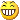Passion Coding！

 本版积分规则 回帖并转播 回帖后跳转到最后一页

GMT+8, 2021-10-22 05:01 , Processed in 0.046887 second(s), 30 queries , Gzip On.